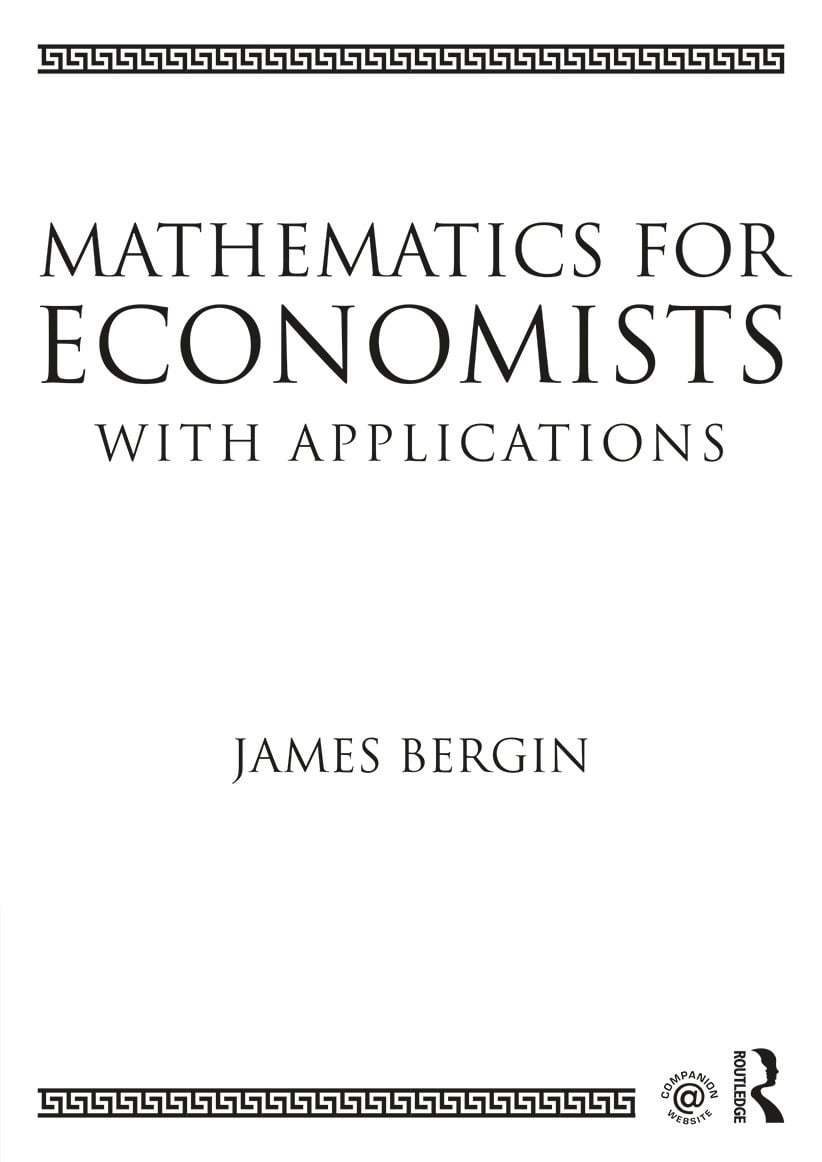# Mathematics for Economists with Applications

## 1st Edition

Routledge

690 pages | 143 B/W Illus.

Paperback: 9780415638289
pub: 2014-12-18
SAVE ~\$23.00
\$115.00
\$92.00
x
Hardback: 9780415638272
pub: 2014-12-18
SAVE ~\$45.00
\$225.00
\$180.00
x
eBook (VitalSource) : 9781315819532
pub: 2015-01-09
from \$57.50

FREE Standard Shipping!

### Description

Mathematics for Economists with Applications provides detailed coverage of the mathematical techniques essential for undergraduate and introductory graduate work in economics, business and finance.

Beginning with linear algebra and matrix theory, the book develops the techniques of univariate and multivariate calculus used in economics, proceeding to discuss the theory of optimization in detail. Integration, differential and difference equations are considered in subsequent chapters. Uniquely, the book also features a discussion of statistics and probability, including a study of the key distributions and their role in hypothesis testing. Throughout the text, large numbers of new and insightful examples and an extensive use of graphs explain and motivate the material. Each chapter develops from an elementary level and builds to more advanced topics, providing logical progression for the student, and enabling instructors to prescribe material to the required level of the course.

With coverage substantial in depth as well as breadth, and including a companion website at www.routledge.com/cw/bergin, containing exercises related to the worked examples from each chapter of the book, Mathematics for Economists with Applications contains everything needed to understand and apply the mathematical methods and practices fundamental to the study of economics.

1. Introduction

2. Matrices and Systems of Equations

3. Linear Algebra: Applications

4. Linear Programming

5. Functions of One Variable

6. Functions of One Variable: Applications

7. Systems of Equations, Differentials and Derivatives

8. Taylor Series

9. Vectors

11. Multivariate Optimization

12. Equality Constrained Optimization

13. Inequality Constrained Optimization

14. Integration

15. Eigenvalues and Eigenvectors

16. Differential Equations

17. Linear Difference Equations

18. Probability and Distributions

19. Estimation and Hypothesis Testing

James Bergin is Professor of Economics at Queen’s University, Canada.

### Subject Categories

BUS000000
BUS021000
BUS044000
BUSINESS & ECONOMICS / Economics / Microeconomics
BUS069000
BUSINESS & ECONOMICS / Economics / General
BUS069030
BUSINESS & ECONOMICS / Economics / Theory
BUS091000
MAT000000
MATHEMATICS / General
MAT002000
MATHEMATICS / Algebra / General
MAT004000
MATHEMATICS / Arithmetic
MAT005000
MATHEMATICS / Calculus
MAT008000
MATHEMATICS / Discrete Mathematics
MAT017000
MATHEMATICS / Linear Programming
MAT029000
MATHEMATICS / Probability & Statistics / General
MAT034000
MATHEMATICS / Mathematical Analysis